Anzeige

# Lec10.pptx

21. Mar 2023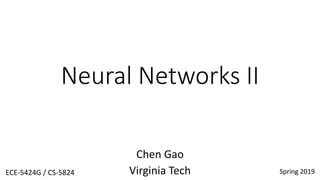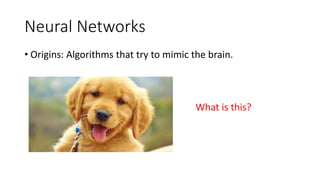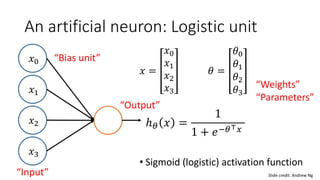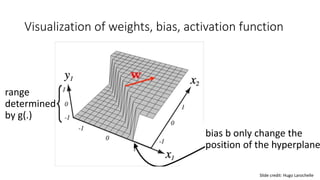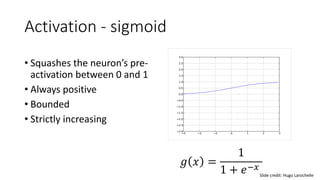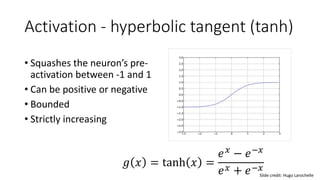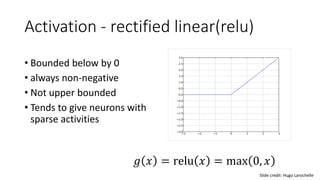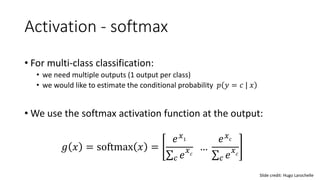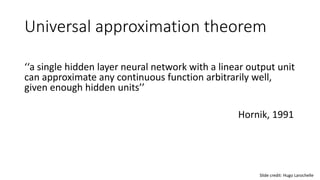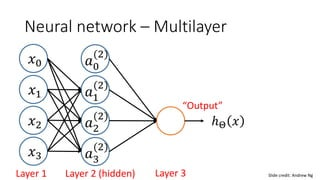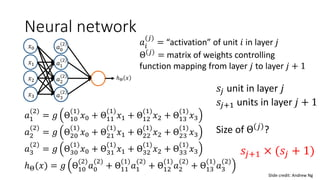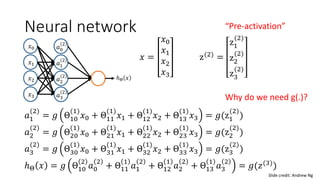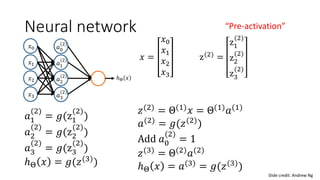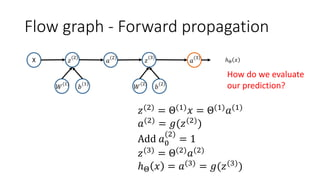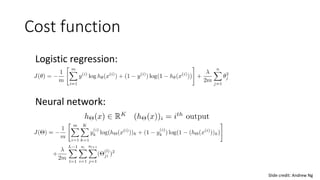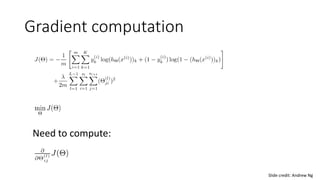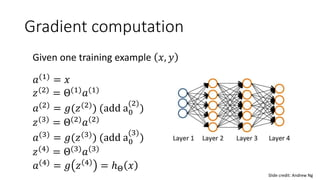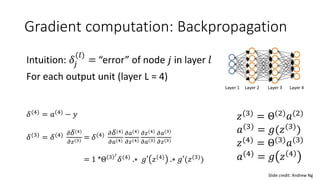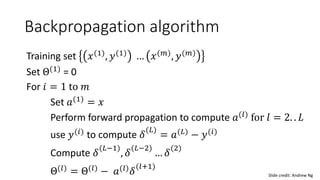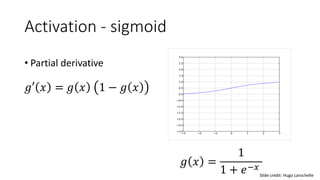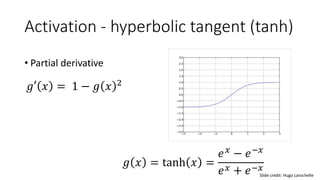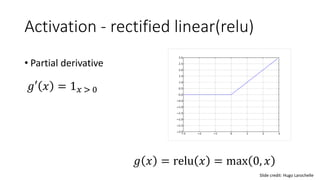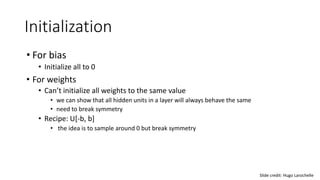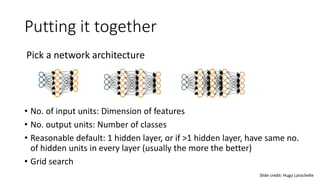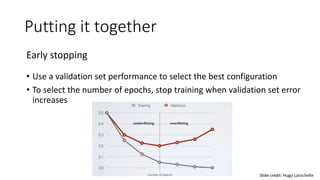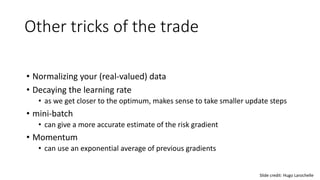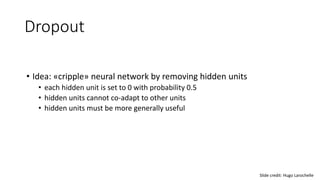1 von 28
Anzeige

### Lec10.pptx

1. Neural Networks II Chen Gao Virginia Tech Spring 2019 ECE-5424G / CS-5824
2. Neural Networks • Origins: Algorithms that try to mimic the brain. What is this?
3. A single neuron in the brain Input Output Slide credit: Andrew Ng
4. An artificial neuron: Logistic unit 𝑥 = 𝑥0 𝑥1 𝑥2 𝑥3 𝜃 = 𝜃0 𝜃1 𝜃2 𝜃3 • Sigmoid (logistic) activation function 𝑥1 𝑥2 𝑥3 𝑥0 ℎ𝜃 𝑥 = 1 1 + 𝑒−𝜃⊤𝑥 “Bias unit” “Input” “Output” “Weights” “Parameters” Slide credit: Andrew Ng
5. Visualization of weights, bias, activation function bias b only change the position of the hyperplane Slide credit: Hugo Larochelle range determined by g(.)
6. Activation - sigmoid • Squashes the neuron’s pre- activation between 0 and 1 • Always positive • Bounded • Strictly increasing 𝑔 𝑥 = 1 1 + 𝑒−𝑥 Slide credit: Hugo Larochelle
7. Activation - hyperbolic tangent (tanh) • Squashes the neuron’s pre- activation between -1 and 1 • Can be positive or negative • Bounded • Strictly increasing 𝑔 𝑥 = tanh 𝑥 = 𝑒𝑥 − 𝑒−𝑥 𝑒𝑥 + 𝑒−𝑥 Slide credit: Hugo Larochelle
8. Activation - rectified linear(relu) • Bounded below by 0 • always non-negative • Not upper bounded • Tends to give neurons with sparse activities Slide credit: Hugo Larochelle 𝑔 𝑥 = relu 𝑥 = max 0, 𝑥
9. Activation - softmax • For multi-class classification: • we need multiple outputs (1 output per class) • we would like to estimate the conditional probability 𝑝 𝑦 = 𝑐 | 𝑥 • We use the softmax activation function at the output: 𝑔 𝑥 = softmax 𝑥 = 𝑒𝑥1 𝑐 𝑒 𝑥𝑐 … 𝑒𝑥𝑐 𝑐 𝑒 𝑥𝑐 Slide credit: Hugo Larochelle
10. Universal approximation theorem ‘‘a single hidden layer neural network with a linear output unit can approximate any continuous function arbitrarily well, given enough hidden units’’ Hornik, 1991 Slide credit: Hugo Larochelle
11. Neural network – Multilayer 𝑎1 (2) 𝑎2 (2) 𝑎3 (2) 𝑎0 (2) ℎΘ 𝑥 Layer 1 “Output” 𝑥1 𝑥2 𝑥3 𝑥0 Layer 2 (hidden) Layer 3 Slide credit: Andrew Ng
12. Neural network 𝑎1 (2) 𝑎2 (2) 𝑎3 (2) 𝑎0 (2) ℎΘ 𝑥 𝑥1 𝑥2 𝑥3 𝑥0 𝑎𝑖 (𝑗) = “activation” of unit 𝑖 in layer 𝑗 Θ 𝑗 = matrix of weights controlling function mapping from layer 𝑗 to layer 𝑗 + 1 𝑎1 (2) = 𝑔 Θ10 (1) 𝑥0 + Θ11 (1) 𝑥1 + Θ12 (1) 𝑥2 + Θ13 (1) 𝑥3 𝑎2 (2) = 𝑔 Θ20 (1) 𝑥0 + Θ21 (1) 𝑥1 + Θ22 (1) 𝑥2 + Θ23 (1) 𝑥3 𝑎3 (2) = 𝑔 Θ30 (1) 𝑥0 + Θ31 (1) 𝑥1 + Θ32 (1) 𝑥2 + Θ33 (1) 𝑥3 ℎΘ(𝑥) = 𝑔 Θ10 (2) 𝑎0 (2) + Θ11 (1) 𝑎1 (2) + Θ12 (1) 𝑎2 (2) + Θ13 (1) 𝑎3 (2) 𝑠𝑗 unit in layer 𝑗 𝑠𝑗+1 units in layer 𝑗 + 1 Size of Θ 𝑗 ? 𝑠𝑗+1 × (𝑠𝑗 + 1) Slide credit: Andrew Ng
13. Neural network 𝑎1 (2) 𝑎2 (2) 𝑎3 (2) 𝑎0 (2) ℎΘ 𝑥 𝑥1 𝑥2 𝑥3 𝑥0 𝑥 = 𝑥0 𝑥1 𝑥2 𝑥3 z(2) = z1 (2) z2 (2) z3 (2) 𝑎1 (2) = 𝑔 Θ10 (1) 𝑥0 + Θ11 (1) 𝑥1 + Θ12 (1) 𝑥2 + Θ13 (1) 𝑥3 = 𝑔(z1 (2) ) 𝑎2 (2) = 𝑔 Θ20 (1) 𝑥0 + Θ21 (1) 𝑥1 + Θ22 (1) 𝑥2 + Θ23 (1) 𝑥3 = 𝑔(z2 (2) ) 𝑎3 (2) = 𝑔 Θ30 (1) 𝑥0 + Θ31 (1) 𝑥1 + Θ32 (1) 𝑥2 + Θ33 (1) 𝑥3 = 𝑔(z3 (2) ) ℎΘ 𝑥 = 𝑔 Θ10 2 𝑎0 2 + Θ11 1 𝑎1 2 + Θ12 1 𝑎2 2 + Θ13 1 𝑎3 2 = 𝑔(𝑧(3)) “Pre-activation” Slide credit: Andrew Ng Why do we need g(.)?
14. Neural network 𝑎1 (2) 𝑎2 (2) 𝑎3 (2) 𝑎0 (2) ℎΘ 𝑥 𝑥1 𝑥2 𝑥3 𝑥0 𝑥 = 𝑥0 𝑥1 𝑥2 𝑥3 z(2) = z1 (2) z2 (2) z3 (2) 𝑎1 (2) = 𝑔(z1 (2) ) 𝑎2 (2) = 𝑔(z2 (2) ) 𝑎3 (2) = 𝑔(z3 (2) ) ℎΘ 𝑥 = 𝑔(𝑧(3) ) “Pre-activation” 𝑧(2) = Θ(1) 𝑥 = Θ(1) 𝑎(1) 𝑎(2) = 𝑔(𝑧(2) ) Add 𝑎0 (2) = 1 𝑧(3) = Θ(2) 𝑎(2) ℎΘ 𝑥 = 𝑎(3) = 𝑔(𝑧(3) ) Slide credit: Andrew Ng
15. Flow graph - Forward propagation 𝑎 (2) 𝑧 (2) 𝑎 (3) ℎΘ 𝑥 X 𝑊 (1) 𝑏 (1) 𝑧 (3) 𝑊 (2) 𝑏 (2) 𝑧(2) = Θ(1) 𝑥 = Θ(1) 𝑎(1) 𝑎(2) = 𝑔(𝑧(2) ) Add 𝑎0 (2) = 1 𝑧(3) = Θ(2) 𝑎(2) ℎΘ 𝑥 = 𝑎(3) = 𝑔(𝑧(3) ) How do we evaluate our prediction?
16. Cost function Logistic regression: Neural network: Slide credit: Andrew Ng
17. Gradient computation Need to compute: Slide credit: Andrew Ng
18. Gradient computation Given one training example 𝑥, 𝑦 𝑎(1) = 𝑥 𝑧(2) = Θ(1) 𝑎(1) 𝑎(2) = 𝑔(𝑧(2) ) (add a0 (2) ) 𝑧(3) = Θ(2) 𝑎(2) 𝑎(3) = 𝑔(𝑧(3) ) (add a0 (3) ) 𝑧(4) = Θ(3) 𝑎(3) 𝑎(4) = 𝑔 𝑧 4 = ℎΘ 𝑥 Slide credit: Andrew Ng
19. Gradient computation: Backpropagation Intuition: ߜ𝑗 (𝑙) = “error” of node 𝑗 in layer 𝑙 For each output unit (layer L = 4) Slide credit: Andrew Ng ߜ(4) = 𝑎(4) − 𝑦 ߜ(3) = ߜ(4) 𝜕ߜ(4) 𝜕𝑧(3) = ߜ(4) 𝜕ߜ(4) 𝜕𝑎(4) 𝜕𝑎(4) 𝜕𝑧(4) 𝜕𝑧(4) 𝜕𝑎(3) 𝜕𝑎(3) 𝜕𝑧(3) = 1 *Θ 3 𝑇 ߜ(4) .∗ 𝑔′ 𝑧 4 .∗ 𝑔′(𝑧(3) ) 𝑧(3) = Θ(2) 𝑎(2) 𝑎(3) = 𝑔(𝑧(3) ) 𝑧(4) = Θ(3) 𝑎(3) 𝑎(4) = 𝑔 𝑧 4
20. Backpropagation algorithm Training set 𝑥(1) , 𝑦(1) … 𝑥(𝑚) , 𝑦(𝑚) Set Θ(1) = 0 For 𝑖 = 1 to 𝑚 Set 𝑎(1) = 𝑥 Perform forward propagation to compute 𝑎(𝑙) for 𝑙 = 2. . 𝐿 use 𝑦(𝑖) to compute ߜ (𝐿) = 𝑎(𝐿) − 𝑦(𝑖) Compute ߜ (𝐿−1) , ߜ (𝐿−2) … ߜ (2) Θ(𝑙) = Θ(𝑙) − 𝑎(𝑙) ߜ (𝑙+1) Slide credit: Andrew Ng
21. Activation - sigmoid • Partial derivative 𝑔 𝑥 = 1 1 + 𝑒−𝑥 Slide credit: Hugo Larochelle 𝑔′ 𝑥 = 𝑔 𝑥 1 − 𝑔 𝑥
22. Activation - hyperbolic tangent (tanh) 𝑔 𝑥 = tanh 𝑥 = 𝑒𝑥 − 𝑒−𝑥 𝑒𝑥 + 𝑒−𝑥 Slide credit: Hugo Larochelle • Partial derivative 𝑔′ 𝑥 = 1 − 𝑔 𝑥 2
23. Activation - rectified linear(relu) Slide credit: Hugo Larochelle 𝑔 𝑥 = relu 𝑥 = max 0, 𝑥 • Partial derivative 𝑔′ 𝑥 = 1𝑥 > 0
24. Initialization • For bias • Initialize all to 0 • For weights • Can’t initialize all weights to the same value • we can show that all hidden units in a layer will always behave the same • need to break symmetry • Recipe: U[-b, b] • the idea is to sample around 0 but break symmetry Slide credit: Hugo Larochelle
25. Putting it together Pick a network architecture Slide credit: Hugo Larochelle • No. of input units: Dimension of features • No. output units: Number of classes • Reasonable default: 1 hidden layer, or if >1 hidden layer, have same no. of hidden units in every layer (usually the more the better) • Grid search
26. Putting it together Early stopping Slide credit: Hugo Larochelle • Use a validation set performance to select the best configuration • To select the number of epochs, stop training when validation set error increases
27. Other tricks of the trade Slide credit: Hugo Larochelle • Normalizing your (real-valued) data • Decaying the learning rate • as we get closer to the optimum, makes sense to take smaller update steps • mini-batch • can give a more accurate estimate of the risk gradient • Momentum • can use an exponential average of previous gradients
28. Dropout Slide credit: Hugo Larochelle • Idea: «cripple» neural network by removing hidden units • each hidden unit is set to 0 with probability 0.5 • hidden units cannot co-adapt to other units • hidden units must be more generally useful

### Hinweis der Redaktion

1. Two ears, two eye, color, coat of hair  puppy Edges, simple pattern  ear/eye Low level feature  high level feature
2. If activated  activation
3. Non-linear
Anzeige